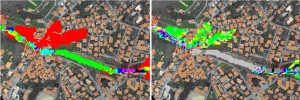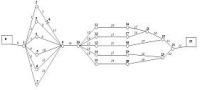Two Seminars have recently been held at the Wessex Institute, presented by Professor Stefano Mambretti of the Politecnico di Milano, Italy.  The first discussed Risk of Flood and Debris Flow and the second Transients in Pipes, Water Hammer Problems.

### Risk of Flood and Debris Flow

Over the years the risk of flooding has increased not only due to climate change, which in many cases has still to be properly quantified, but also because of high city development, which is not always respectful of the environment.

Risk R is defined as convolution between the two functions hazard H and vulnerability V, multiplied for the exposition E.

R = H • V • E

Hydraulic engineering tries to define the hazard of a flood event as a combination of depth and velocity of the water; mathematical models help in the assessment of these values.

Mathematical models can be one, two or three-dimensional.

One-dimensional models are nowadays widespread and their use is well assessed. However, only in a few occasions are they useful for hazard assessment. One case was referred to during the seminar, when a flood prone area was studied in order to decide when it should be evacuated, as no protection was feasible. In this case the hazard cannot be reduced, but the risk can be relieved by reducing the vulnerability of the houses and the exposition of people.

Two-dimensional models are becoming more popular because of the increase in the computational power and the improvements in the mathematical models. With these models it is actually possible to estimate the depth and the velocity of the flood wave on a flood prone area.Flood prone area and the results of the simulation: depth (left) and velocity (right)

On the other hand, three-dimensional models are based on Reynolds equations (a simplified form of the Navier-Stokes equation) where some empirical models of turbulence have to be used. So far, the promising advantages which could be reached using a more sophisticated model are neglected by the uncertainties which must be introduced and the overwhelming computational effort required.

Many schemes have been developed for integrating the so-called shallow water equations (or two-dimensional De Saint Venant equations) to describe two dimensional flow. MacCormak and Lax-Wendroff second order schemes and Lax-Friedrichs first order schemes have been tried and compared. Lax-Friedrichs, despite probably being less accurate, is more stable and much faster, and therefore it has been tested against laboratory data, obtaining satisfactory results.Laboratory reproduction of a debris flow, which is then analysed with a specifically developed software
The final target of the research is the implementation of a two-phase and two-dimensional model to simulate the debris flow phenomena. Debris flows are a solid-liquid mixture: water, clay, and larger solid material (sand, gravel, and rocks), with characteristics between clear water flow and a landslide.

So far, the different phases of debris flow (triggering, propagation and deposition) are modelled separately, with different approaches. Most methodologies, moreover, are empirical and do not produce good results.

The research tends towards a comprehensive approach of this phenomenon.

### Transients in Pipes, Water Hammer Problems

There are a number of examples of benefits derived by the presence of unsteady flow. For instance, the 'Hydraulic ram', invented by a Swiss clockmaker in 1772, which is a cyclic water pump powered by hydropower. It requires no outside source of power other than the kinetic energy of water and therefore it is sometimes used in remote areas.

However, in most cases this phenomenon has destructive characteristics and, despite having been studied for many years, many uncertainties are still present when a complex model system is built and the peak values of the pressures (positive and negative) need to be damped.

The analysis of a complex plant is carried out by means of numerical integration of the governing equations, a set of hyperbolic differential equations. These equations are well known and their integration has been performed by means of the method of characteristics since the mid 1960s.From the real plant...
Unfortunately, when the pressure drops close to absolute zero (p = –1 atm referring to atmospheric pressure), there is an increase in the dimensions of the air nuclei present in the water and the flow becomes multiphase, for which the characteristics are determined by the resistance forces and by the slope of the pipe. The presence of the air nuclei smooth the pressures due to the transient and reduces the wave celerity. Classical models produce results which cannot be considered to be reliable....the numerical model is built...
Improving one dimensional models imply the insertion of new terms to compute the
headlosses, as one of the main problems in solving waterhammer problems is related to the use of steady flow formulas.

To evaluate the resistances in case of unsteady flow a number of approaches are possible which can be of increasing complexity from the simple consideration of variations of average velocity to the most complex models which include second derivatives of the...and the results are analysed.
velocities both in the longitudinal and in the axial directions.

The improvement of one dimensional models with the insertion of these new terms for evaluating the resistances produces reasonable results until water column separation is reached. In this latter case, 1D models fail.

However, smoothing and phase shift are present even when no air release occurs, that implies that the problems are probably tied to the unsteady flow phenomena; the reason for such inadequacy of 1D models  has to be found on the different velocity profiles between those in unsteady flow and the corresponding ones (i.e. with the same discharge) in steady flow. In unsteady flow the gradient of the velocity close to the pipe wall is steeper than in steady flow and therefore the stresses are higher, and finally so are the resistances.

Therefore, by means of a number of experimental tests, the aim of the research is the development of a two dimensional model which is able to predict the pressures in case of depressions and water column separation.  As these simulations are time demanding and they are also difficult to implement, the research of an appropriate parameter to decide when to “switch” from the simpler, and faster, one-dimensional and one-phase model to the more complex one is necessary.